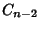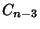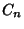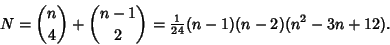## Diagonal (Polygon)

A Line Segment connecting two nonadjacent Vertices of a Polygon. The number of ways a fixed convex-gon can be divided into Triangles by nonintersecting diagonals is(withdiagonals), whereis a Catalan Number. This is Euler's Polygon Division Problem. Counting the number of regions determined by drawing the diagonals of a regular-gon is a more difficult problem, as is determining the number of-tuples of Concurrent diagonals (Beeler et al. 1972, Item 2).

The number of regions which the diagonals of a Convex Polygon divide its center if no three are concurrent in its interior isThe first few values are 0, 0, 1, 4, 11, 25, 50, 91, 154, 246, ... (Sloane's A006522).

See also Catalan Number, Diagonal (Polyhedron), Euler's Polygon Division Problem, Polygon, Vertex (Polygon)

References

Beeler, M.; Gosper, R. W.; and Schroeppel, R. HAKMEM. Cambridge, MA: MIT Artificial Intelligence Laboratory, Memo AIM-239, Feb. 1972.

Sloane, N. J. A. Sequence A006522/M3413 in An On-Line Version of the Encyclopedia of Integer Sequences.'' http://www.research.att.com/~njas/sequences/eisonline.html and Sloane, N. J. A. and Plouffe, S. The Encyclopedia of Integer Sequences. San Diego: Academic Press, 1995.